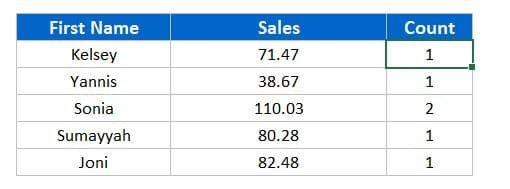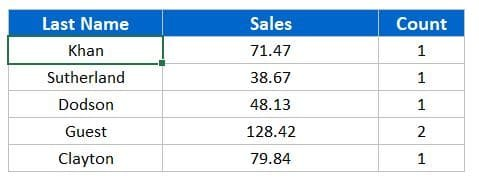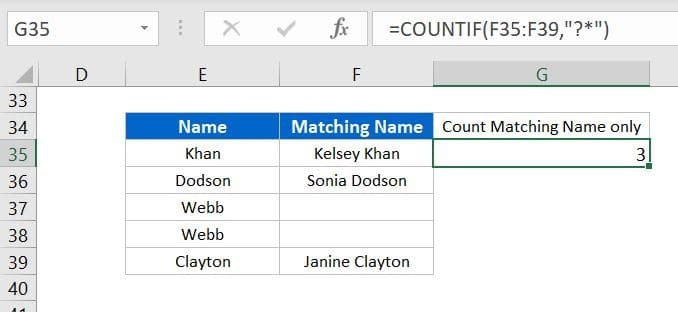# Wildcard in Microsoft Excel

In this article, you will learn how to use Wildcard in Microsoft Excel. Wildcard is very useful in excel and can make your work very easy.

## What is Wildcard?

Wildcards are basically special characters. Using wildcards you can perform a fuzzy matching. They can take the place of any characters.

There are 3 types of wildcards available in Excel. They are asterisk (*), the question mark (?), and the tilde (~).

### Below is the few Example of using these wildcards:

WildcardHow to workExamples
?Single text character"A", "B", "a", "b", etc.
??Double text Character"PK","xy","im" etc.
?*One or greater than one character"a", "pk", "Raj", "XYZ", etc.
??*Two or greater than two character"ab", "pk", "Jack", "Sunday", etc.
(???)3 Characters in the brackets"(Sun)","(Mon)",(Xyz)" etc.
*For any text character"pk", "PK", "abc123", etc.
*tionEnds in "tion""Condition","Objection", "Tradition" etc.
pk*Starts with "pk""PK's Chart", "pk's dashboards","PK's youtube Channel" etc.
*PK*Contains "PK""PK's Chart","Who is PK?", "Me and PK are working together" etc.
*~?Ends in question mark"How are you?" etc.
~**Starts with "*""* T&C applied" etc.

## Where can we use wildcards in Excel?

We can use wildcards in 3 places in excel – Formulas, Filters and Find & Replace

## Wildcards in Formulas:

Below are the most common formulas wherein we use the wildcards in Excel:

Examples: Below are the below examples for wildcards in the formulas

We have some sample data as given in below image-

Example-1: To get the Sum of Sales and Count of full name on the base of First name, we have use below formulas-

Formula for Sales-

`=SUMIF(A:A,F4&"*",C:C)`

Formula for Count-

`=COUNTIF(A:A,F4&"*")`Sum of Sales and Count of full name on the base of First name

Example-2: To get the Sum of Sales and Count of full name on the base of Last name, we have use below formulas-

Formula for Sales-

`=SUMIF(A:A,"*"&F11,C:C)`

Formula for Count-

`=COUNTIF(A:A,"*"&F11)`Sum of Sales and Count of full name on the base of Last name

Example-3: We can use wildcards with VLOOKUP function also. To get the Short Name on the base of last name we have used below given formula-

`=VLOOKUP("*"&F18,A:B,2,0)`

Example-4: To get the count of only text values from a range, we can use “*” wildcard-

`=COUNTIF(E27:E31,"*")`

Example-5: To get the count of text wherein at-least one character is available.

`=COUNTIF(F35:F39,"?*")`Count of text wherein at-least one character is available

Example-6: There are few other examples where we have used wildcard in below image-

### Count of Short name wherein it has number of character given in cell L3

`=COUNTIF(B2:B22,REPT("?",L3))`

### Sum of Sales wherein Short Name has number of character given in cell L3

`=SUMIF(B2:B22,REPT("?",L3),C2:C22)`

### Count where STD Code is two digits long in the Text column. There are few phone numbers with STD codes available in the Text Column like – “+1234-564875”, “+11-65976548”,”+33-56468651” etc.

`=COUNTIF(D2:D22,"+??-*")`

### Count of the Questions in the Text Column

=COUNTIF(D2:D22,”*~?”)

### Count start with * in the Text Column

`=COUNTIF(D2:D22,"~**")`

### Count where 3 character are in brackets the Text Column

`=COUNTIF(D2:D22,"(???)")`

## Wildcards in Filters:

You can use the wildcards while using the filter in Excel. Below are the below examples-

To filter all the Full Name starting with “K”, you can put “k*” in the search box-

To filter all the questions from the Text Column you put “~?” in the search box-

## Wildcards in Find and Replace:

We can use the wildcard in Find and Replace also. Below are the few examples-

We can separate the Employee Name and Emp Id from the combination of Employee Name and ID which it together with “-” delimiter

To get the only Names from Name and Emp ID, we replace “-*” with blank

Only Name will be available-

To get the only Emp IDs from Name and Emp ID, we replace “*-” with blank